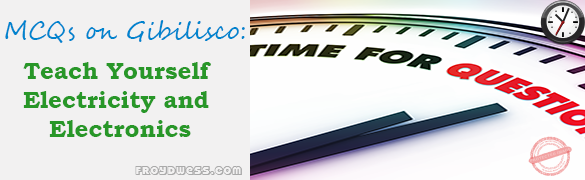# Power and Resonance in AC circuits – Gibilisco MCQs in Electronics

(Last Updated On: December 8, 2017)This is the Multiple Choice Questions (MCQs) in Chapter 17: Power and Resonance in AC circuits from the book Teach Yourself Electricity and Electronics, 5th edition by Stan Gibilisco. If you are looking for a reviewer in Electronics Engineering this will definitely help you before taking the Board Exam.

### Start Practice Exam Test Questions

1. The power in a reactance is:

• A. Radiated power.
• B. True power.
• C. Imaginary power.
• D. Apparent power.

2. Which of the following is not an example of true power?

• A. Power that heats a resistor.
• B. Power radiated from an antenna.
• C. Power in a capacitor.
• D. Heat loss in a feed line.

3. The apparent power in a circuit is 100 watts, and the imaginary power is 40 watts. The true power is:

• A. 92 watts.
• B. 100 watts.
• C. 140 watts.
• D. Not determinable from this information.

4. Power factor is equal to:

• A. Apparent power divided by true power.
• B. Imaginary power divided by apparent power.
• C. Imaginary power divided by true power.
• D. True power divided by apparent power.

5. A circuit has a resistance of 300 W and an inductance of 13.5 μH in series at 10.0 MHz. What is the power factor?

• A. 0.334.
• B. 0.999.
• C. 0.595.
• D. It can’t be found from the data given.

6. A series circuit has Z = 88.4 Ω with R = 50.0 Ω What is PF?

• A. 99.9 percent.
• B. 56.6 percent.
• C. 60.5 percent.
• D. 29.5 percent.

7. A series circuit has R = 53.5 Ω and X = 75.5 Ω. What is PF?

• A. 70.9 percent.
• B. 81.6 percent.
• C. 57.8 percent.
• D. 63.2 percent.

8. Phase angle is equal to:

• A. Arctan Z/R.
• B. Arctan R/Z.
• C. Arctan R/X.
• D. Arctan X/R.

9. A wattmeter shows 220 watts of VA power in a circuit. There is a resistance of 50 Ω in series with a capacitive reactance of −20 Ω. What is the true power?

• A. 237 watts.
• B. 204 watts.
• C. 88.0 watts.
• D. 81.6 watts.

10. A wattmeter shows 57 watts of VA power in a circuit. The resistance is known to be 50 Ω, and the true power is known to be 40 watts. What is the absolute-value impedance?

• A. 50 Ω
• B. 57 Ω
• C. 71 Ω
• D. It can’t be calculated from this data.

11. Which of the following is the most important consideration in a transmission line?

• A. The characteristic impedance.
• B. The resistance.
• C. Minimizing the loss.
• D. The VA power.

12. Which of the following does not increase the loss in a transmission line?

• A. Reducing the power output of the source.
• B. Increasing the degree of mismatch between the line and the load.
• C. Reducing the diameter of the line conductors.
• D. Raising the frequency.

13. A problem that standing waves can cause is:

• A. Feed line overheating.
• B. Excessive power loss.
• C. Inaccuracy in power measurement.
• D. All of the above.

14. A coil and capacitor are in series. The inductance is 88 mH and the capacitance is 1000 pF. What is the resonant frequency?

• A. 17 kHz.
• B. 540 Hz.
• C. 17 MHz.
• D. 540 kHz.

15. A coil and capacitor are in parallel, with L = 10.0 μH and C = 10 pF. What is fo?

• A. 15.9 kHz.
• B. 5.04 MHz.
• C. 15.9 MHz.
• D. 50.4 MHz.

16. A series-resonant circuit is to be made for 14.1 MHz. A coil of 13.5 μH is available. What size capacitor is needed?

• A. 0.945 μF.
• B. 9.45 pF.
• C. 94.5 pF.
• D. 945 pF.

17. A parallel-resonant circuit is to be made for 21.3 MHz. A capacitor of 22.0 pF is available. What size coil is needed?

• A. 2.54 mH.
• B. 254 μH.
• C. 25.4 μH.
• D. 2.54 μH.

18. A 1/4-wave line section is made for 21.1 MHz, using cable with a velocity factor of 0.800. How many meters long is it?

• A. 11.1 m.
• B. 3.55 m.
• C. 8.87 m.
• D. 2.84 m.

19. The fourth harmonic of 800 kHz is:

• A. 200 kHz.
• B. 400 kHz.
• C. 3.20 MHz.
• D. 4.00 MHz.

20. How long is a 1/2-wave dipole for 3.60 MHz?

• A. 130 feet.
• B. 1680 feet.
• C. 39.7 feet.
• D. 515 feet.

Rate this: